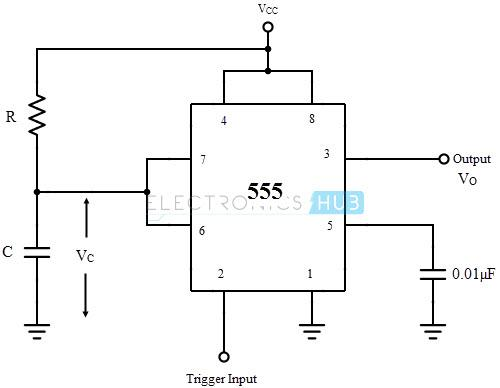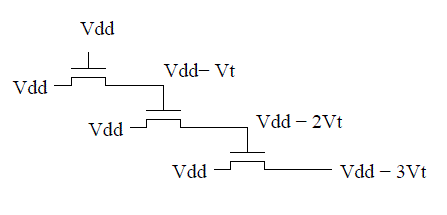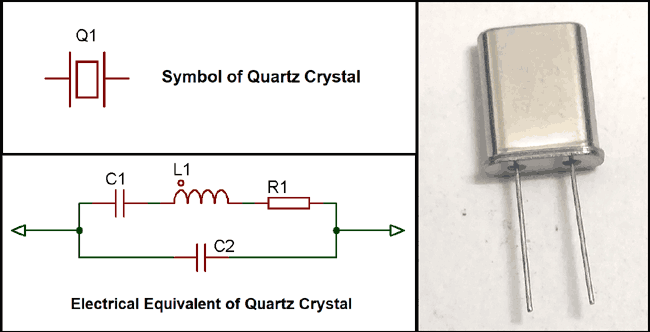### Que.91 What is a monostable multivibrator?

• It is a circuit whose output has one stable state and one quasi-stable state, if external input is not applied then it remains in the stable state forever.
• If the trigger input is applied, then the output goes into a quasi-stable state but again returns to a stable state after a short duration of time. Thus a monostable ckt. generates pulse output when the trigger is applied.
•### Que. 93 What is a pass transistor?

• It is a MOSFET used as a switch to pass logic levels between nodes of a circuit instead of a switch connected directly to a supply voltage.
•• When the gate voltage is equal to (source) input voltage, switch ON and the output becomes equal to (Vdd – Vt).
• When the input voltage is zero, the output voltage is zero. The switch is OFF.
• ### Que.44 What is the early effect or base width modulation in BJT? What are its consequences?

• It refers to the decrease in effective base width due to a decrease in reverse bias voltage across the collector junction.
• The early effect has three consequences:
• Large signal current (alpha) increase- as Vcb increases effective base width decrease which implies a decrease in no. of recombination or increase in collector current, hence alpha increases.
• For small increases in alpha, beta(small-signal current gain) increases by a large amount.
• Emitter current Ie increases due to an increase in hole concentration gradient.
• ### Que.80 Types of audio-frequency amplifiers and their application.

Answer: Audio frequency oscillator circuits are used in the audio systems to generate audible sound. For example, doorbells, and alarm systems.

There are two types of audio frequency oscillators:

• Wein bridge oscillator: it consist of a noninverting amplifier that provides zero phase shift and an RC feedback network that also provides zero phase shift. Hence phase shift remains zero and oscillation occur at frequency fo. These are mostly used for audio testing.
• RC phase shift oscillator: which can be made using either BJT, FET, or OP-AMP. These oscillators are used in musical instruments, GPS units, and voice synthesis.
• ### Que.53 What are the applications of current mirror circuits?

Application of current mirror circuits:

• A current mirror is used as a constant current source to bias the BJT in IC amplifiers.
• A current mirror is used as an active load in ICs amplifiers to obtain higher voltage gain.
• ### Que.76 What is a Crystal oscillator? What is the application of a crystal oscillator?

Answer: It is an LC oscillator in which a quartz crystal is used in place of the inductor.

• Quartz is a piezoelectric material that converts energy from electrical to mechanical and mechanical to electrical. The circuit diagram includes R, L, and C as components of quartz material in parallel with a capacitor.
•Application of crystal oscillator:

• crystal oscillators are used when a stable frequency is needed such as to generate a clock signal for the microprocessor.
• All electronic watches use a crystal oscillator.

• Maximum power can be transferred to the load due to the impedence matching property of the transformer.
• Dc isolation between amplifier and load.
• Better efficiency because primary winding does not dissipate DC power.
• Higher voltage gain than RC coupled amplifier if turns ratio is greater than 2.
• The disadvantage of transformer-coupled amplifier:

• Improper frequency response.
• The transformer is bigger in size and costlier.
• Application of transformer-coupled amplifier:

• These are used in power amplifiers.